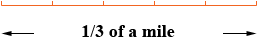What is 2/3 divided by 5? Thank You, KH Hi, Let me start with one-third rather than two-thirds. Suppose that you have 1/3 of a mile and you want to divide it by 5, that is divide it into 5 equal lengths.It takes 5 of these equal lengths to make 1/3 of a mile and hence it takes 53 = 15 such lengths to make one mile. Thus each of these lengths is one-fifteenth of a mile. Thus 1/3 divided by 5 is 1/15. Hence 2/3 divided by 5 is 2/15. Penny